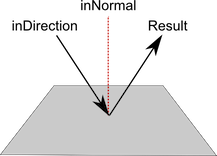Version: 2017.3
언어: 한국어

Vector3.Reflect

매뉴얼로 전환
public static Vector3 Reflect (Vector3 inDirection, Vector3 inNormal);

설명

Reflects a vector off the plane defined by a normal.

The inNormal vector defines a plane (a plane's normal is the vector that is perpendicular to its surface). the inDirection vector is treated as a directional arrow coming in to the plane. The returned value is a vector of equal magnitude to inDirection but with its direction reflected.Reflection of a vector off a plane.

using UnityEngine;
using System.Collections;

public class ExampleClass : MonoBehaviour { public Transform originalObject; public Transform reflectedObject; void Update() { reflectedObject.position = Vector3.Reflect(originalObject.position, Vector3.right); } }